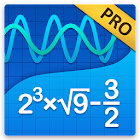Graphing Calculator + Math PRO

• SEARCH TYPE

All Android applications categories

All Android games categories# Graphing Calculator + Math PRO

5.9K 8.3

8.3 Expert
rating

## Graphing Calculator + Math PRO's review

Published: 2013-10-08, by Peter Warrior.

## A comprehensive scientific calculator app

8.3
• It does whatever you may need
• Smooth UI
• No complaints

## "Rocket scientists wanted"

Yes, your droid comes with a calculator and you might even already have an additional calculator app. However, if you are surviving high school or are in any scientific degree in college, those toy calculators aren't enough. You need to solve quadratic equations and polynomials and vectors and matrixes and draw graphs and all that odd Math stuff we mere mortals can't understand. Perhaps you need to save it all and bring it with you to your PC.

Graphing Calculator is an insanely complete app, thought for helping any need any algebra student may need. It also comes with a smooth interface and a supporting website and email address.

This pro version comes free of ads and can be run offline.

Tags: ?????????? ??? ????????by Peter , Appszoom

Oct 08, 2013

Description

If you're looking for a graphing calculator app that works smoothly and seamlessly, you've found it! Graphing Calculator by Mathlab is a scientific graphing calculator integrated with algebra and is an indispensable mathematical tool for students in elementary school to those in college or graduate school, or just anyone who needs more than what a basic calculator offers. It is designed to replace bulky and costly handheld graphing calculators and works on virtually any Android phone or tablet.

Furthermore, Graphing Calculator by Mathlab displays calculations as it performs them on the high-quality display of the Android device, making it easier for the user to understand the calculations and see them clearly. This app has two great strengths. First, it acts as a fine scientific calculator, but, more than that, it displays the intermediate results of the calculations as you type. It allows the students to both watch and learn how the calculations are made and how to find the final answer. Second, the graphing ability is absolutely stunning! Not only does the calculator beautifully display the graphs, but it automatically generates the x- and y- values and displays them as well.

Video: https://youtu.be/6BR8Lv1U9kA
Help site with instructions and examples: http://help.mathlab.us
If you have a question, send email to calc@mathlab.us

PRO FEATURES
* Full screen graphs
* 9 workspaces
* Longer input and history
* Save constants, functions and expressions in the library
* Physical constants
* Internet is not required
* 30 days refund

SCIENTIFIC CALCULATOR
* Square root, cube and higher roots (hold √ key)
* Exponent or power, use x^ key, (x^2)
* Logarithms ln(), log(), log[base]()
* Trigonometric functions sin π/2, cos 30°, ...
* Hyperbolic functions sinh, cosh, tanh, ... (hold "e" key to switch)
* Inverse functions (hold direct function key)
* Complex numbers, all functions support complex arguments
* Derivatives sin x' = cos x, ... (hold x^ key)
* Scientific and engineering notation (enable in menu)
* Percent mode
* Binary, octal and hexadecimal numbers, 0b1010, 0o123, 0xABC

GRAPHING CALCULATOR
* Multiple functions graphing
* Implicit functions up to 2nd degree (ellipse 2x^2+3y^2=1, etc.)
* Polar graphs (r=cos2θ)
* Parametric functions, enter each on new line (x=cos t, y=sin t)
* Function roots and critical points.
* Graph intersections
* Tracing function values and slopes
* Slide to scroll
* Pinch to zoom
* Fullscreen graphs (PRO)
* Function tables
* Save graphs as images
* Save tables as csv
* 3D graphs

FRACTION CALCULATOR
* Simple and complex fractions 1/2 + 1/3 = 5/6
* Mixed numbers, use space to enter values 3 1/2
* Use parentheses (1+2)/(3+4)=3/7

ALGEBRA CALCULATOR
* Linear equations x+1=2 -> x=1
* Quadratic equations x^2-1=0 -> x=-1,1
* Approximate roots of higher polynomials
* Systems of linear equations, write one equation per line, x1+x2=1, x1-x2=2
* Polynomial long division
* Polynomial expansion, factoring

PROBABILITY AND STATISTICS
* Hold n! key to enter functions, double tap dot to enter comma
* Combinations nCr(5,2)=10
* Permutations nPr(5,2)=20
* Sample A=[1 2 3 4 5]
* Sample B=[2 3 4 5 6]
* Sum sum(A) = 15
* Average avg(A) = 3
* Median median(A) = 3
* Sample variance var(A) = 2.5
* Population variance varp(A) = 2
* Standard deviation stdev(A) = 1.58..., stdevp(A) = 1.41...
* Covariance cov(A,B) = 2.5
* Correlation corr(A,B) = 1

MATRIX CALCULATOR
* Matrix and vector arithmetic operations
* Vector cross product, dot product (hold *) and norm
* Matrix determinant, inverse, transpose and trace functions

LIBRARY
* Physical constants (PRO)
* User defined constants and functions (PRO)
* Save expressions for future reference

## Users review

from 5.879 reviews

"Awesome"

9.6